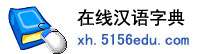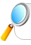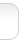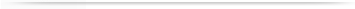汉字   按部首检索  按拼音检索涂资 饶资 贾资 鲁资 应资 伍资 闫资 赵资 谢资

 资字的男姓名 | 资字的女姓名 | 资开头的姓名 | 资结尾的姓名 | 更多带资的姓名
 资博 (男) 资 (男) 资 (女) 天资 (女) 资龙 (男) 贞资 (男) 资程 (男) 资平 (男) 资颖 (女) 家资 (男) 资源 (男) 资涵 (男) 资江 (男) 小资 (女) 资婷 (女) 资勇 (男) 资丰 (男) 资明 (女) 资英 (女) 天资 (男) 俊资 (女) 彦资 (女) 资兑 (男) 资刚 (男) 资伟 (男) 资林 (男) 资华 (男) 资吟 (女) 资翼 (女) 资衡 (男) 资民 (男) 传资 (男) 资尧 (男) 资金 (女) 益资 (男) 资历 (男) 资沅 (男) 资样 (男) 资政 (女) 树资 (男) 资桦 (女) 资锋 (男) 资兴 (男) 资家 (男) 元资 (男) 雪资 (女) 资旦 (男) 灭资 (女) 殷资 (男) 资娟 (女) 蓄资 (男) 资庆 (男) 资丽 (女) 资颖 (男) 灭资 (男) 资福 (男) 文资 (男) 钦资 (女) 资阳 (女) 资远 (男) 国资 (男) 翔资 (男) 光资 (男) 本资 (男) 高资 (男) 玉资 (男) 涤资 (男) 婷资 (女) 资君 (女) 资玮 (女) 资君 (男) 资广 (男) 资敏 (女) 资廷 (男) 资丹 (女) 洪资 (男) 运资 (男) 资泳 (男) 资凯 (男) 克资 (男) 资廷 (女) 资委 (男) 资海 (男) 良资 (男) 资荣 (男) 资连 (男) 资杨 (男) 资琴 (女) 资茂 (男) 英资 (女) 资明 (男) 资贵 (男) 资娇 (女) 经资 (男) 资燕 (女) 资超 (女) 邱资 (女) 沛资 (男) 资文 (女) 新资 (男) 资智 (男) 远资 (男) 资超 (男) 资阳 (男) 资勤 (男) 幸资 (男) 资生 (男) 革资 (男) 学资 (男) 资杰 (男) 资山 (男) 资程 (女) 延资 (男) 西资 (男) 资斌 (男) 翼资 (男) 资鑫 (男) 资倩 (男)

 版权所有　在线汉语字典   浙ICP备05019169号